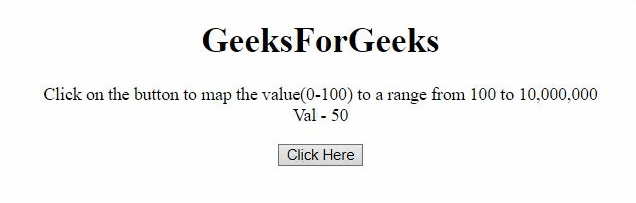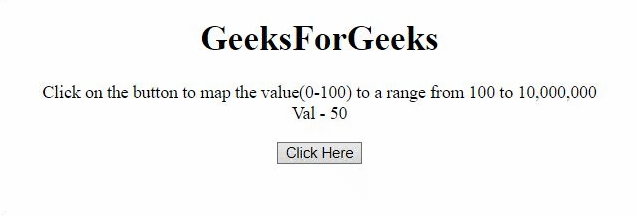Related Articles

# How to create slider to map a range of values in JavaScript ?

• Last Updated : 13 Jul, 2020

The task is to map a range of values to another range of values like map (0-100) to (100-10000000) in JavaScript. There are two approaches that are discussed below.

Approach 1: Use the logarithmic scale to map the range. In this example, first the log value of the range is calculated and then the scale is calculated using the difference of log values of target scale to the difference of start scale. Then use the exponentiation to map the range for a particular number.

Example: This example implements the above approach.

 ```<``html``>`` ` `<``body` `style``=``"text-align:center;"``>``    ``<``h1``>GeeksForGeeks``     ` `    ``<``p` `id``=``"GFG_UP"``>``     ` `    ``<``button` `onclick``=``"myGFG()"``>``        ``Click Here``    ````     ` `    ``<``p` `id``=``"GFG_DOWN"``>``    ``<``script``>``        ``var up = document.getElementById("GFG_UP");``        ``var val = 50;``        ``up.innerHTML = "Click on the button to map"``            ``+ " the value(0-100) to a range from "``            ``+ "100 to 10, 000, 000<``br``> Val - " + val;``         ` `        ``var down = document.getElementById("GFG_DOWN");``         ` `        ``function Slider(pos) {``            ``var minM = 0;``            ``var maxM = 100;``            ``var minV = Math.log(100);``            ``var maxV = Math.log(10000000);``            ``var scal = (maxV - minV) / (maxM - minM);``            ``return Math.exp(minV + scal * (pos - minM));``        ``}``        ``function myGFG() {``            ``down.innerHTML = "The value for " ``                ``+ val + " is " + Slider(val);``        ``}``    `````` ` ``

Output:Approach 2: This example also maps the value from one range to another but uses a different formula.

Example: This example implements the above approach.

 ```<``html``>`` ` `<``body` `style``=``"text-align:center;"``>``    ``<``h1``>GeeksForGeeks`` ` `    ``<``p` `id``=``"GFG_UP"``>``     ` `    ``<``button` `onclick``=``"myGFG()"``>``        ``Click Here``    ````     ` `    ``<``p` `id``=``"GFG_DOWN"``>``     ` `    ``<``script``>``        ``var up = document.getElementById("GFG_UP");``        ``var val = 50;``        ``up.innerHTML = "Click on the button to map"``            ``+ " the value(0-100) to a range from "``            ``+ "100 to 10, 000, 000<``br``> Val - " + val;`` ` `        ``var down = document.getElementById("GFG_DOWN");``        ``function Slider(pos) {``            ``return Math.floor(-900 + 1000 * ``                    ``Math.exp(pos / 10.857255959));``        ``}``        ``function myGFG() {``            ``down.innerHTML = "The value for " ``            ``+ val + " is " + Slider(val);``        ``}``    `````` ` ``

Output:Hey geek! The constant emerging technologies in the world of web development always keeps the excitement for this subject through the roof. But before you tackle the big projects, we suggest you start by learning the basics. Kickstart your web development journey by learning JS concepts with our JavaScript Course. Now at it’s lowest price ever!

My Personal Notes arrow_drop_up